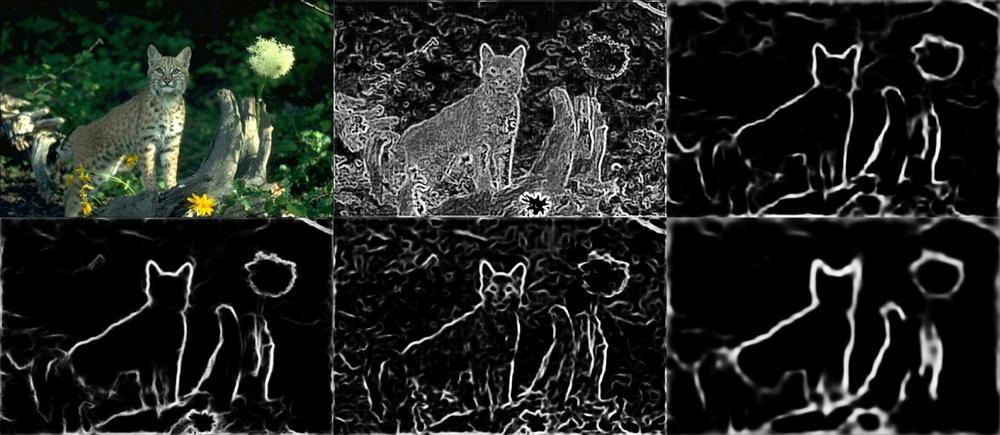## canny 算子

canny算法是一种multi-stage 的算法，其处理图片的过程分为如下五个步骤：

1. Noise Reduction

2. Finding Intensity Gradient of the Image

3.Non-Maximun Suppression

4.Double Threhold

5.Edge tracking by hysteresis

python实现代码如下：

``````import cv2
import numpy as np
import matplotlib.pyplot as plt

edges = cv2.Canny(img,100,200) #参数:图片，minval，maxval,kernel = 3

plt.subplot(121) #121表示行数，列数，图片的序号即共一行两列，第一张图
plt.imshow(img,cmap='gray') #cmap :colormap 设置颜色
plt.title('original image'),plt.xticks([]),plt.yticks([]) #坐标轴起点，终点的值
plt.subplot(122),plt.imshow(edges,cmap = 'gray')
plt.title('edge image'),plt.xticks([]),plt.yticks([])

plt.show()``````

## Fast Edge Detection Using Structured Forests

``````import cv2
import matplotlib.pyplot as plt
import numpy as np

filename = 'img/doge.jpg'
cur_path = "E:/workSpace/AI/edgeDetection/"
model_path = cur_path + "resources/model.yml.gz"
if image.size == 0:
exit(0)
img = np.float32(image)
img = img*(1.0/255.0)
retval = cv2.ximgproc.createStructuredEdgeDetection(model_path)
a = retval.detectEdges(img)
plt.subplot(121)
plt.imshow(image,cmap='gray')
plt.subplot(122)
plt.imshow(a,cmap= 'gray')
plt.show()``````

## Holistically-Nested Edge Detection（HED）

• Holistically ：表示边缘预测结果是基于端对端的
• Nested：生成的Edge过程中，将许多尺度不同的图片进行fusion得到结果。

## 算法比较

canny算子，由算法步骤可以看出canny算子更注重像素梯度变化所体现的出来的边缘信息，不考虑实际物体，同时损失了图片的空间信息，对于一些边缘颜色与背景颜色相近的图片，很可能会丢失掉边缘信息。

canny处理边缘颜色与背景颜色相似的图片效果：

Fast edge算法使用随机森林来生成边缘信息，针对图片窗口（patch）区域进行边缘的提取，利用ground true，能够体现实际的物体，可以反映出图片的空间信息。

HED使用VGG改造的网络，针对整张图片提取特征信息，通过多尺度融合，multi-loss等方式。同样可以反映边缘的特征信息。效果如下：